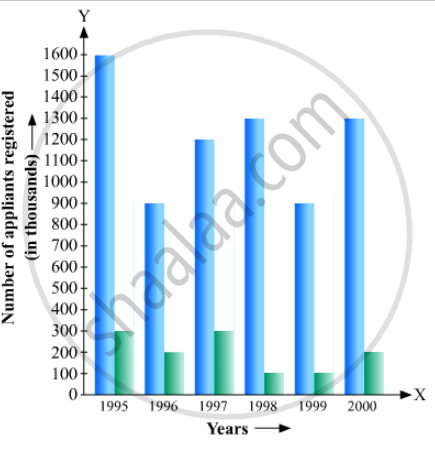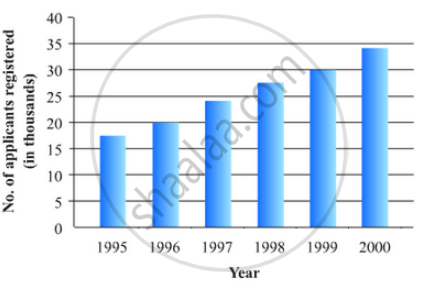# The Following Data Gives the Number (In Thousands) of Applicants Registered with an Construct a Bar Graph to Represent the Above Data. - Mathematics

The following data gives the number (in thousands) of applicants registered with an

 Year 1995 1996 1997 1998 1999 2000 Number of applicants registered (in thousands) 18 20 24 28 30 34

Construct a bar graph to represent the above data.#### Solution

To represent the given data by a vertical bar graph, we first draw horizontal and vertical axes. Let us consider that the horizontal and vertical axes represent the years and the number of applicants registered in thousands respectively. We have to draw 6 bars of different lengths given in the table.

At first we mark 6 points in the horizontal axis at equal distances and erect rectangles of the same width at these points. The heights of the rectangles are proportional to the number of applicants registered.

The vertical bar graph of the given data is following:Concept: Graphical Representation of Data
Is there an error in this question or solution?

#### APPEARS IN

RD Sharma Mathematics for Class 9
Chapter 23 Graphical Representation of Statistical Data
Exercise 23.2 | Q 4 | Page 25

Share